# Pipeline Connectivity-Functional Multiplex Comparison WU

Description:

This is the Graphical User Interface (GUI) tutorial for the pipeline that compares two groups of subjects using connectivity and functional multiplex data (such as DWI and fMRI) and weighted undirected graphs.

• Start BRAPH 2 and select the Pipeline from the main GUI

Start MATLAB, navigate to the BRAPH2 folder and run “braph2” with the following command:

>> braph2

Select the Pipeline Connectivity-Functional Multiplex Comparison WU in the right menu. You can use the search field, typing connectivity functional for example (Figure 1).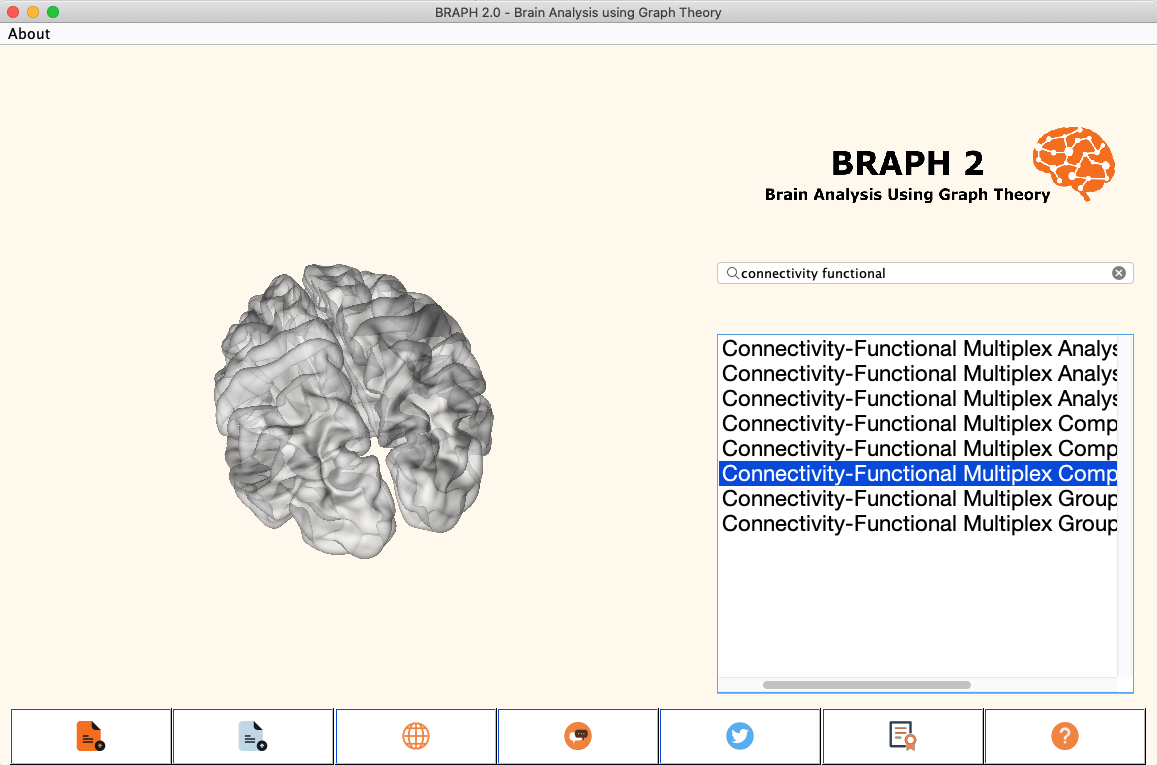Figure 1. Landing GUI.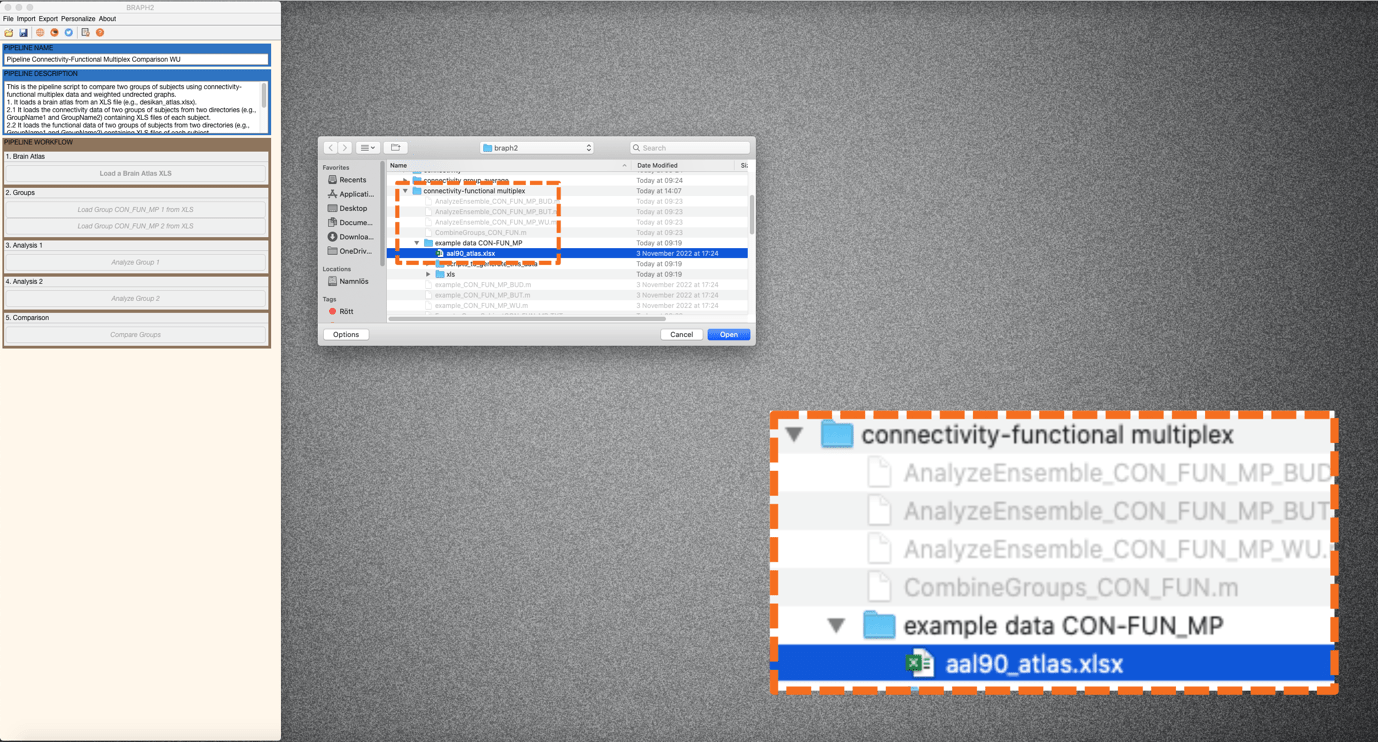The first step is to load a brain atlas from an XLS file. Press the button “Load a Brain Atlas XLS” (the only one active in the pipeline GUI, Figure 2) and then select the aal90_atlas.xlsx in the directory ./braph2/pipelines/connectivity-functional multiplex/example data CON-FUN_MP (Figure 2). Finally, to visualize the atlas press “Plot the brain atlas” and play with the surface settings if desired. Check the Brain Atlas section from the tutorial of module 1 for more information on how to control the appearance in this interface.

First load the data of group 1 by pressing “Load Group CON FUN MP 1 from XLS”. Then, a user request figure will appear asking you to upload the connectivity data, press “Ok” and then select the folder of Groupname1 inside the Connectivity folder (in the directory ./braph2/pipelines/connectivity-functional multiplex/example data CON-FUN_MP/xls/connectivity/GroupName1 (Figure 3). Once connectivity data for group 1 is selected, then again a user request figure will appear asking now to upload the functional data, press “Ok” and then select the folder of Groupname1 inside the Functional folder (in the directory ./braph2/pipelines/connectivity-functional multiplex/example data CON-FUN_MP/xls/functional/GroupName1 (Figure 4).

After, load the data for the group 2 which can be found in the directory ./braph2/pipelines/connectivity-functional multiplex/example data CON-FUN_MP/xls/connectivity/GroupName2 for connectivity the data and in the directory ./braph2/pipelines/connectivity-functional multiplex/example data CON-FUN_MP/xls/functional/GroupName2 for the functional data.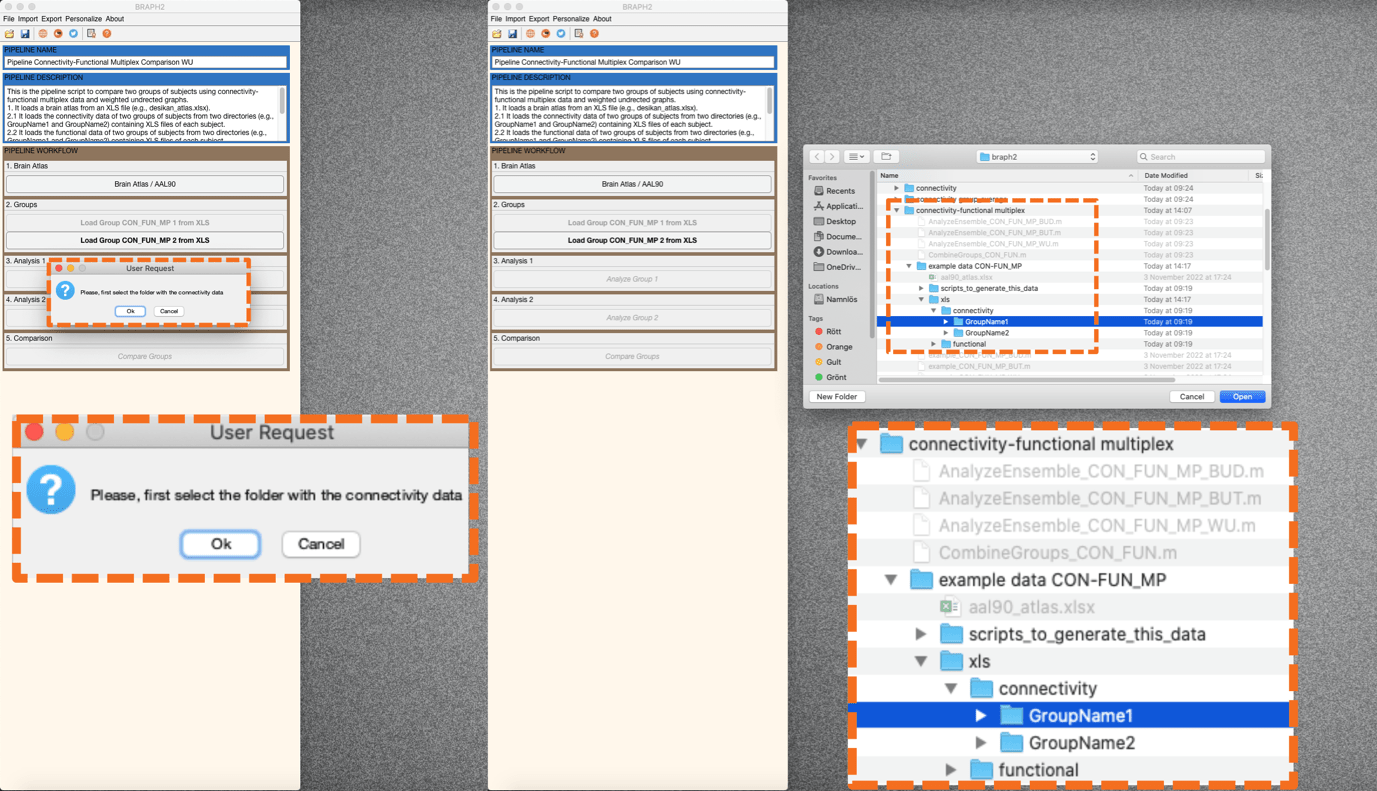Figure 3. First load the connectivity data for group 1 (GroupName1).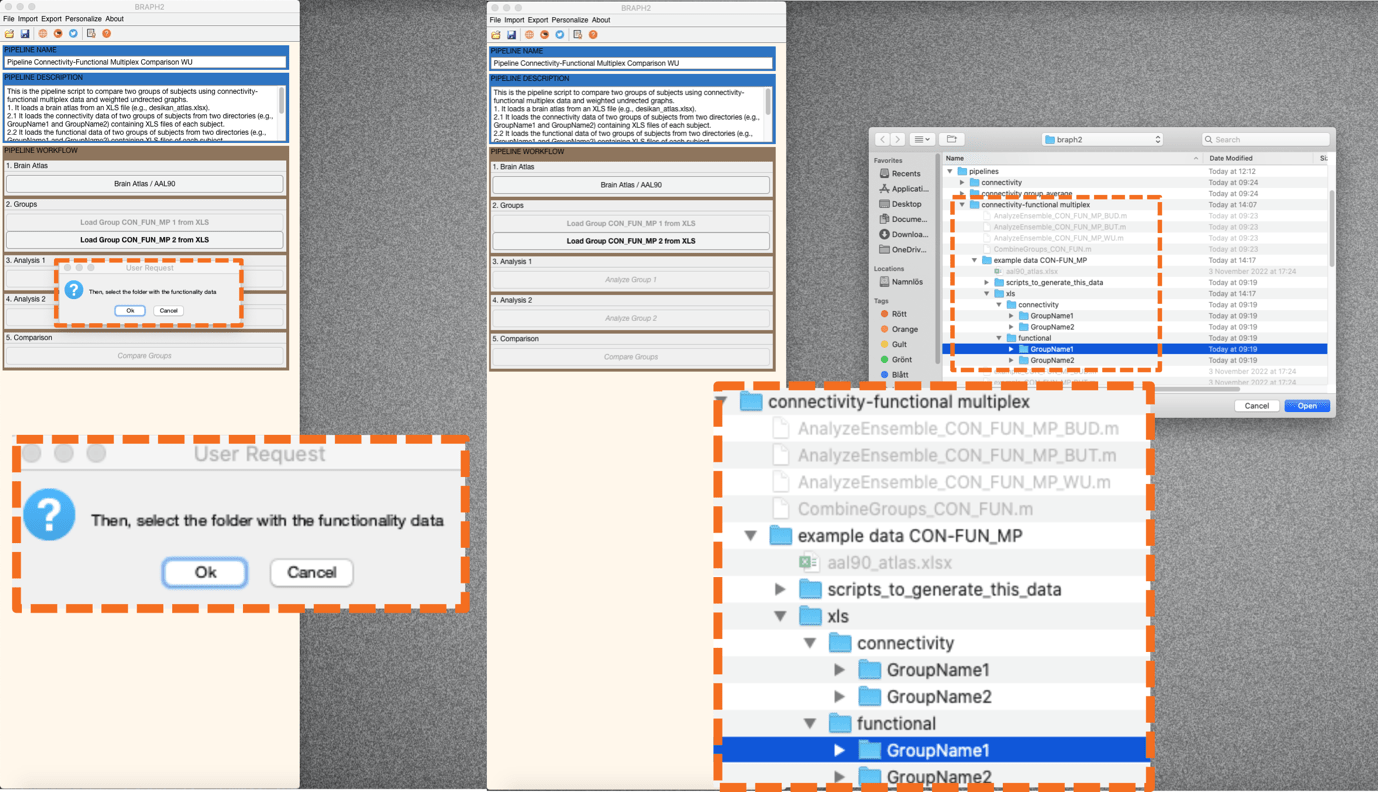Figure 4. Second load the functional data for group 1 (GroupName1).

• Performing the connectivity-functional multiplex analysis of group 1

Analysis of the first group using connectivity-functional multiplex analyses (CON-FUN) based on weighted undirected graphs (WU).

First press “Analyze Group 1” to open the interface for the analysis, where you can specify the parameters for constructing the graph from the time series of functional data (type of correlation, and what to do with the negative weights). Then under the GRAPHS section, by pressing “C” you can check the graphs for each subject. More interesting is to plot the average graph for the whole group; to do this go to section GRAPH MEASURES and press “C”, right click on the left of a measure to deploy the menu seen on Figure 5 and select “Plot Graph”. We will see an adjacency graph on the right, which is the graph from the first layer (the connectivity data layer, see Figure 5) and from the first subject. If we go to settings and then to section “G”, we can select to plot the Mean Graph for the group instead of the individual graphs. Moreover, if we change to layer 2 then we will see the adjacency matrix from the functional layer (Figure 6).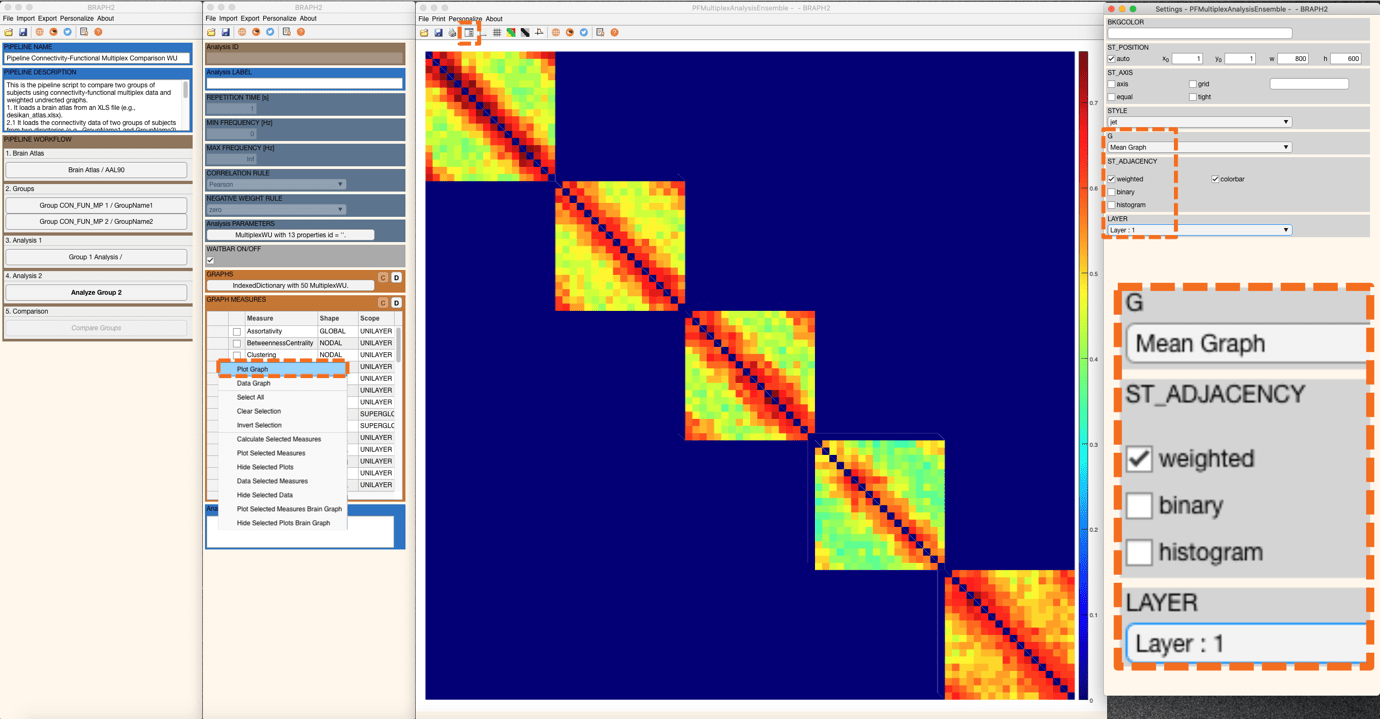Figure 5. Plot graph. By default, we see the adjacency matrix at the first layer, coming from the connectivity data.Figure 6. Plot graph. Adjacency matrix at the second layer, coming from the functional data.

Now it is the moment to calculate some connectivity measures for group 1. Select one measure, for example, Overlapping Strength (nodal superglobal) and Overlapping Strength Average (global superglobal)  and calculate them by right clicking on the left-side of the measures list and press “Calculate Selected Measures” (Figure 7).Figure 7. Calculation of Overlapping Strength and Overlapping Strength Average.

When the measure is calculated, a C appears on its left. Now we just keep selected the Overlapping Strength measure and right click on the left-side of the measures list and press “Data Selected Measures” to be able to check the results of the measure for group 1. From there, we press “Plot Brain Multiplex Graph” to visualize the results of Overlapping Strength for group 1 on a brain surface (Figure 8).

A new figure with the brain surface will open with default settings for brain region visualization. If we go to settings and then scroll down in the Settings panel, we will find Measures and EDGES. By checking the boxes, the connection links will appear, and the size of all spheres will be adapted in proportion to the measure’s value, where blueish colors represent smaller values, and red larger (Figure 8).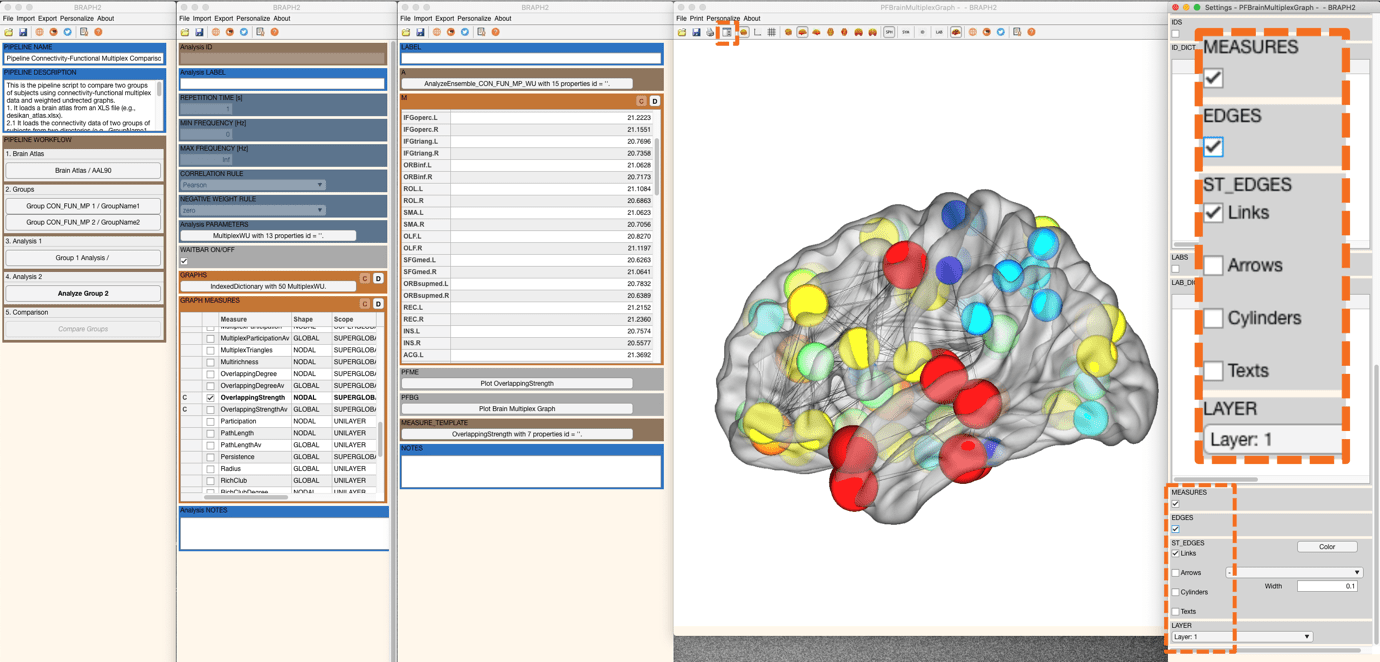Figure 8. Selecting the check box at Measures and Edges.

Remember, in case we want to calculate a measure with different rules or parameters, this is done by going first to “Data Selected Measuresbefore calculating the measure. For more details, check the section Measures’ calculation with different parameters in the tutorial from Module 1.

• Performing the functional analysis of group 2

Analysis of the second group using the same parameters selected for the first group (connectivity-functional multiplex analyses (CON-FUN) based on weighted undirected graphs (WU)).

Follow the previous steps in the analysis of group 1 and explore some other measures for group 2.

• Comparing the two groups

To proceed with the group comparison press “Compare Groups” and the interface to select the comparison parameters will open. After setting the desired permutation number (recommended 1000 – 10000 permutations, although in this tutorial we use 100 for time constraints), press “C” under the ENSEMBLE COMPARISONS section to open the list of measures to use to compare the groups.

We select the Weighted Multiplex Participation measure to be compared, and then right click on the left of the measure table and select “Calculate Selected Comparisons” (Figure 9). Depending on how many permutations you use it will take less or more time.Figure 9. Calculation of Group Comparison.

Once the calculations are done, you should see a C on the left of the measure to be compared. Then press “Data Selected Comparison” to show the difference values between groups and the associated p-values resulting from the permutation testing (Figure 10). Significant differences after FDR correction at the value of q = 0.05 (q level can be changed in the QVALUE) are highlighted in the table by green. We changed the q value to 0.03 and there are still many regions with significant difference in the weighted multiplex participation measure (Figure 10).

We can then plot the results of this nodal measure from the comparison by pressing “Plot Brain Multiplex Graph Comparison” in the measure’s table GUI. A new figure with the brain surface will open with default settings for brain region visualization. If we scroll down in the Settings panel, we will find MEASURES, FDR SHOW, and QVAL. By checking the boxes and changing the QVAL to 0.03 just the regions that are significantly different between groups after applying FDR correction at the desired level will be shown, and the rest will be hidden (Figure 10). In this case, all differences are in the same direction, weighted multiplex participation is larger in group 2 than in group 1 (red color indicates group 2 > group 1).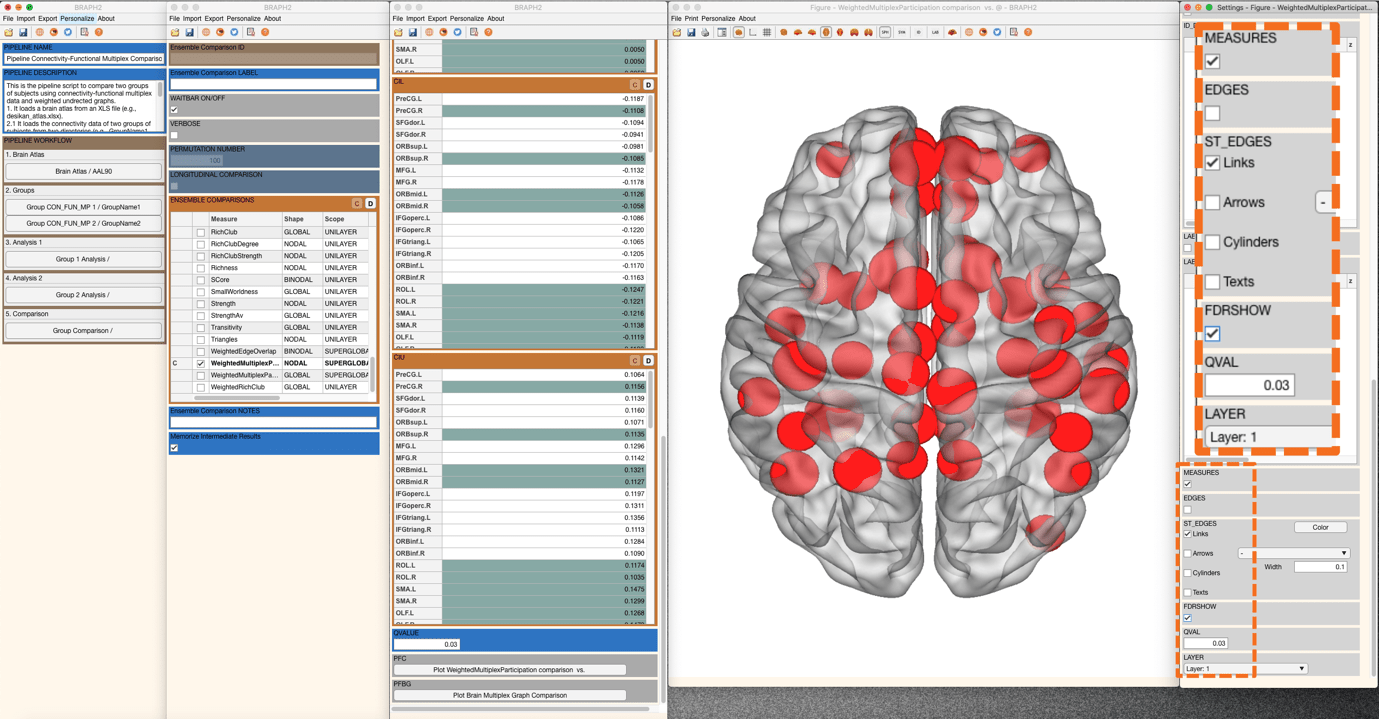Figure 10. Regions that are significant after applying FDR at a q_value = 0.03.